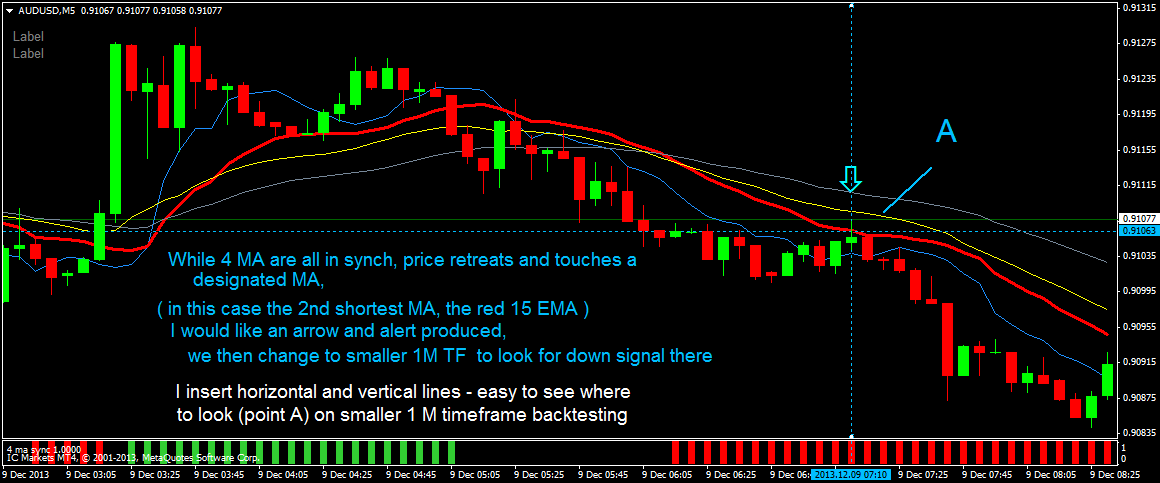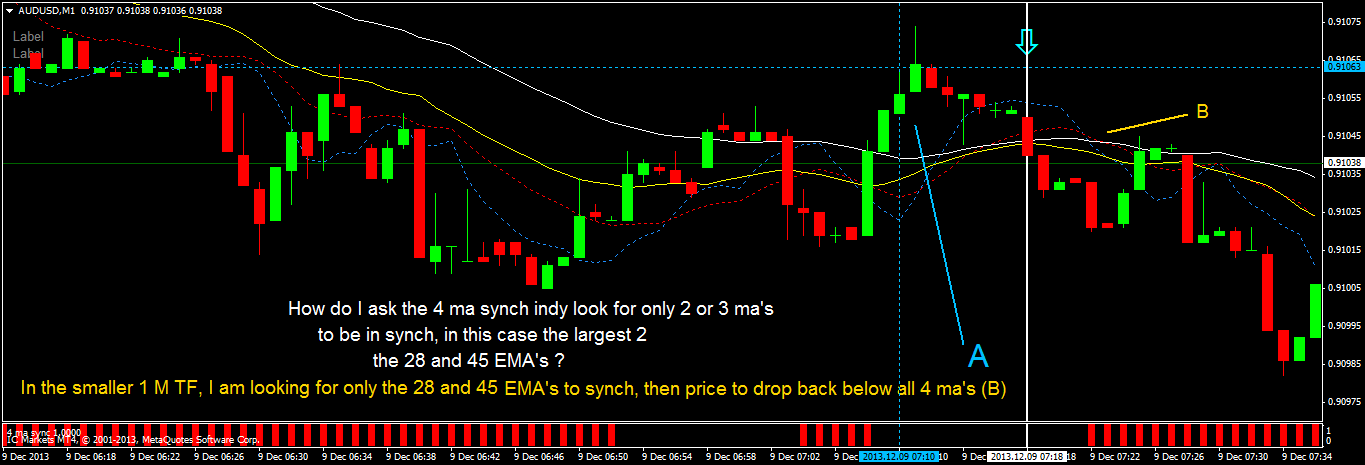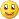# Moving Average - page 105154429

person77:
Fantastic – works very well mladen! Thank you so much.

A couple of requests if it wouldn’t be too much trouble:

1. While 4 MA are all in synch, price retreats and touches a designated MA

( in this case the 2nd shortest MA, the red 15 EMA ) I would like an arrow and alert produced.

I would then change to smaller 1M time frame to look for short signal there.

I insert horizontal and vertical lines so its easy to spot where to look (point A) on smaller 1 M timeframe backtesting2. Can I ask the 4 ma synch indy to only look for two or three ma’s to be in synch in order to show the histogram bar? (please see the 1m conditions attachment for what I am trying to do here)person77

I am afraid that this indicator is not designed or predicted to show price touches. Also I am quite sure that thera are already good indicators that are doing exactly that sort of job.

As of 2 and 3 mas version : simply replace the "" in the code where you see comparison that goes like "if (ma1<ma2 && ma2<3" ... and so on, with "=" and then set 2 mas to same values for 3 different mas or 2 pairs of mas to same values to have 2 mas shown by the indicator47

Thanks for the suggestion.

My coding is next to useless!

I changed the > and < to = but got some errors when compiling.

What do I need to do differently?

//------------------------------------------------------------------

//------------------------------------------------------------------

#property indicator_separate_window

#property indicator_buffers 2

#property indicator_color1 LimeGreen

#property indicator_color2 DarkOrange

#property indicator_width1 2

#property indicator_width2 2

#property indicator_minimum 0

#property indicator_maximum 1

extern int Ma1Period = 5;

extern int Ma1Price = PRICE_CLOSE;

extern int Ma1Method = MODE_EMA;

extern int Ma1Shift = 0;

extern int Ma2Period = 15;

extern int Ma2Price = PRICE_CLOSE;

extern int Ma2Method = MODE_EMA;

extern int Ma2Shift = 0;

extern int Ma3Period = 28;

extern int Ma3Price = PRICE_CLOSE;

extern int Ma3Method = MODE_EMA;

extern int Ma3Shift = 0;

extern int Ma4Period = 45;

extern int Ma4Price = PRICE_CLOSE;

extern int Ma4Method = MODE_EMA;

extern int Ma4Shift = 0;

//

//

//

//

//

double histu[];

double histd[];

//------------------------------------------------------------------

//

//------------------------------------------------------------------

//

//

//

//

//

int init()

{

SetIndexBuffer(0,histu); SetIndexStyle(0,DRAW_HISTOGRAM);

SetIndexBuffer(1,histd); SetIndexStyle(1,DRAW_HISTOGRAM);

return(0);

}

int deinit() { return(0); }

//------------------------------------------------------------------

//

//------------------------------------------------------------------

//

//

//

//

//

int start()

{

int counted_bars = IndicatorCounted();

if (counted_bars < 0) return(-1);

if (counted_bars > 0) counted_bars--;

int limit = MathMin(Bars-counted_bars,Bars-1);

//

//

//

//

//

for(int i = limit; i >=0; i--)

{

double ma1 = iMA(NULL,0,Ma1Period,Ma1Shift,Ma1Method,Ma1Price,i);

double ma2 = iMA(NULL,0,Ma2Period,Ma2Shift,Ma2Method,Ma2Price,i);

double ma3 = iMA(NULL,0,Ma3Period,Ma3Shift,Ma3Method,Ma3Price,i);

double ma4 = iMA(NULL,0,Ma4Period,Ma4Shift,Ma4Method,Ma4Price,i);

histu = EMPTY_VALUE;

histd = EMPTY_VALUE;

if (ma1=ma2 && ma2=ma3 && ma3>ma4) histu = 1;

if (ma1=ma2 && ma2=ma3 && ma3<ma4) histd = 1;

}

return(0);

}13

hi

what about renko chart 2min using MA?154429

person77:

Thanks for the suggestion.

My coding is next to useless!

I changed the > and < to = but got some errors when compiling.

What do I need to do differently?

//------------------------------------------------------------------

//------------------------------------------------------------------

#property indicator_separate_window

#property indicator_buffers 2

#property indicator_color1 LimeGreen

#property indicator_color2 DarkOrange

#property indicator_width1 2

#property indicator_width2 2

#property indicator_minimum 0

#property indicator_maximum 1

extern int Ma1Period = 5;

extern int Ma1Price = PRICE_CLOSE;

extern int Ma1Method = MODE_EMA;

extern int Ma1Shift = 0;

extern int Ma2Period = 15;

extern int Ma2Price = PRICE_CLOSE;

extern int Ma2Method = MODE_EMA;

extern int Ma2Shift = 0;

extern int Ma3Period = 28;

extern int Ma3Price = PRICE_CLOSE;

extern int Ma3Method = MODE_EMA;

extern int Ma3Shift = 0;

extern int Ma4Period = 45;

extern int Ma4Price = PRICE_CLOSE;

extern int Ma4Method = MODE_EMA;

extern int Ma4Shift = 0;

//

//

//

//

//

double histu[];

double histd[];

//------------------------------------------------------------------

//

//------------------------------------------------------------------

//

//

//

//

//

int init()

{

SetIndexBuffer(0,histu); SetIndexStyle(0,DRAW_HISTOGRAM);

SetIndexBuffer(1,histd); SetIndexStyle(1,DRAW_HISTOGRAM);

return(0);

}

int deinit() { return(0); }

//------------------------------------------------------------------

//

//------------------------------------------------------------------

//

//

//

//

//

int start()

{

int counted_bars = IndicatorCounted();

if (counted_bars < 0) return(-1);

if (counted_bars > 0) counted_bars--;

int limit = MathMin(Bars-counted_bars,Bars-1);

//

//

//

//

//

for(int i = limit; i >=0; i--)

{

double ma1 = iMA(NULL,0,Ma1Period,Ma1Shift,Ma1Method,Ma1Price,i);

double ma2 = iMA(NULL,0,Ma2Period,Ma2Shift,Ma2Method,Ma2Price,i);

double ma3 = iMA(NULL,0,Ma3Period,Ma3Shift,Ma3Method,Ma3Price,i);

double ma4 = iMA(NULL,0,Ma4Period,Ma4Shift,Ma4Method,Ma4Price,i);

histu = EMPTY_VALUE;

histd = EMPTY_VALUE;

if (ma1=ma2 && ma2=ma3 && ma3>ma4) histu = 1;

if (ma1=ma2 && ma2=ma3 && ma3<ma4) histd = 1;

}

return(0);

}

person77

You should have replaced "<" with "" with ">=" (not just "=")

_____________________

PS: forgot to tell that 2 MAs are always in sync47

That works perfectly!2047

Kaufman Adaptive moving average (by Alexander Gettinger)

Kaufman Adaptive Moving Average (KAMA) was created by Perry Kaufman and first presented in his book Smarter Trading (1995).

Formulas:

KAMA = KAMA+sc*(Price-KAMA), where

sc = (er*0.6015+0.0645)*(er*0.6015+0.0645),

er = Abs(Price-Price)/Sum1,

Sum1 = Sum(Abs(Price-Price)) from (i-Length+1) to i.

Files:
kama.mq4 2 kb2047

Two versions of linear regression (from the same author)

Files:154429

Kaufman AMA pre-filtered

Preparing Kaufman AMA (adaptive moving average) for some other things, and here is one version that has a very simplified way of using (ama calculation is made a function now). It can have price per-filtering too (instead of using raw price, filtered price can be used (to turn the filtering of, set the PriceFilter to 1)

Files:
kaufman.gif 36 kb2047

MA28 (by Alexander Gettinger)

Files:
ma28.mq4 4 kb
ma28.png 83 kb37

would, please, be possible to make the indicator multitimeframe? thank